Aptitude Tests 4 Me

Non Verbal Logic

Detailed solution

68.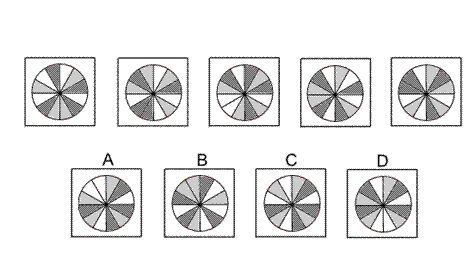Solution: A Explanation: In this question there is a circle divided up into twelve sectors - 4 coloured white, 4 coloured light grey, and 4 coloured dark grey. There are two rules to follow that are applied simultaneously. The first rule is that the circle is rotated by 30° clockwise each time. The second rule is that the colours of the sectors change from one diagram to the next - white changes to light grey, light grey changes to dark grey, and dark grey changes to white. When these two rules are applied simultaneously, the next diagram in the sequence is A.

69.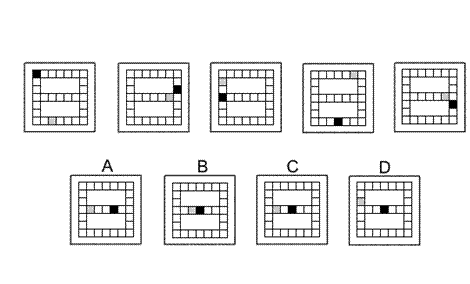Solution: C Explanation: In this question there is a grid in the shape of the number 8 and two squares - one coloured black and one coloured grey. The two squares move around the grid tracing out the number 8 shape as shown by the arrows in the diagram. They move eight squares the first time, seven squares the second time, six the third time, and so on. Following this rule, the next diagram in the sequence must be C.

70.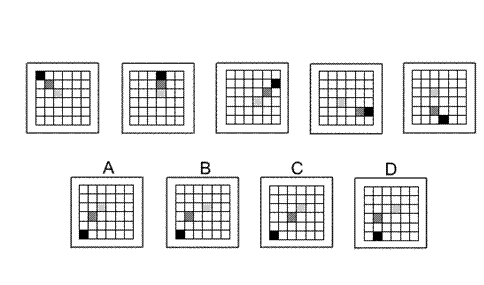Solution: B Explanation: In this question there is a grid of squares, three of which are coloured - one black, one dark grey and one light grey. Each coloured square moves around the grid according to its own rule. The black square moves around an outer circuit of the grid, consisting of all the squares around the perimeter of the grid, and moves three spaces clockwise each time. The light grey square moves around an inner circuit of the grid, consisting of the four squares at the centre of the grid, and moves one space clockwise each time. The dark grey square moves around a middle circuit of the grid, consisting of all the rest of the squares that are not in the outer circuit or the inner circuit, and moves two spaces clockwise each time. When these rules are applied, the next diagram of the sequence is B.

71.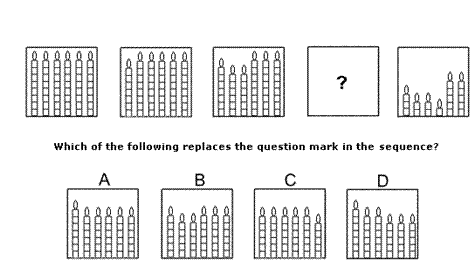Solution: D Explanation: In this question there are six candles each divided into eight equal segments. From the first diagram to the second, the first candle burns one segment. From the second diagram to the third, the next two candles burn two segments each. From the third diagram to the fourth, the next three candles burn three segments each. This gives the correct answer, D. Then from the fourth diagram (D) to the fifth diagram, the next four candles (starting from the beginning again) burn four segments each.

72.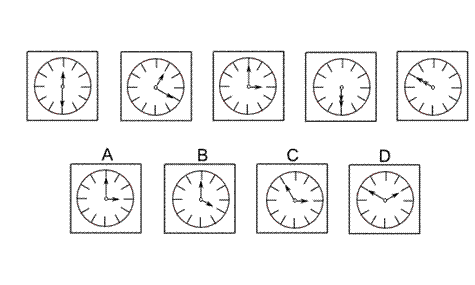Solution: A Explanation: In this question there is a clock face with a long hand and a short hand. The two hands rotate around the centre of the clock each according to its own rule. The short hand rotates clockwise by 30° the first time, 60° the second time, 90° the third time etc. Therefore for the next diagram in the sequence, the short hand should rotate 150° clockwise to point towards the 3 on the clock. The correct answer, therefore, could be A or C. The long hand rotates anticlockwise by 60° the first time, 120° the second time, 180° the third time etc. Therefore for the next diagram in the sequence, the long hand should rotate 300° anticlockwise to point towards the 12 on the clock. The correct answer, therefore, is A.

73.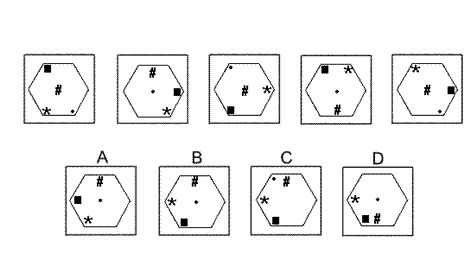Solution: B Explanation: In this question there are four shapes - a dot, a square, an asterisk (*) and a hash symbol (#) - that move around the inside of a hexagon, each according to its own rule. The dot moves along the diagonal of the hexagon that stretches from the top left corner to the bottom right corner, moving backwards and forwards one place at a time. Following this rule, the correct answer could be A, B or D. The square moves around the inside of the vertices of the hexagon, rotating through 120° clockwise each time. Following this rule also, the correct answer must be B or D. The asterisk moves around the inside of the vertices of the hexagon, rotating through 60° anticlockwise each time. Following this rule, the answer is still either B or D. The hash symbol moves up and down the vertical axis of the hexagon, moving one place up or down each time. When all these rules are applied, for the next diagram of the sequence, the correct answer is B.

74.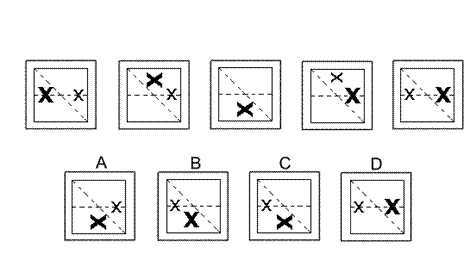Solution: C Explanation: In this question there are two X's, one small and one large, that are reflected alternately in each of two mirror lines - a horizontal line and a diagonal line, as shown. The first time, the small X is reflected in the horizontal line and the big X is reflected in the diagonal line. The second time, the small X is reflected in the diagonal line and the big X is reflected in the horizontal line (and the two X's, in this case, lie one on top of the other - so only the big X is visible), etc. For the next diagram of the sequence, therefore, the small X should be reflected in the horizontal line and the big X should be reflected in the diagonal line, and the correct answer is C.

75.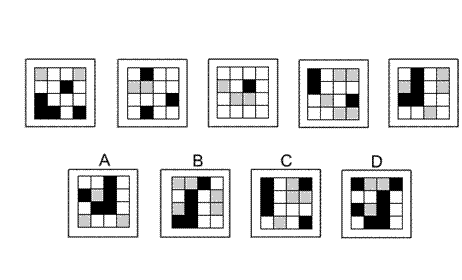Solution: D Explanation: In this question there is a grid of black, grey and white squares. From one diagram to the next, the nine squares in the top left hand corner of one diagram are identical to the nine squares in the bottom right hand corner of the next diagram; so there has been a translation of one square to the right and one square down. Following this rule, the backwards L shape should move one square to the right and one square down, and the correct answer is D.

76.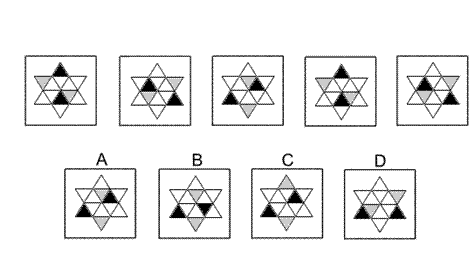Solution: A Explanation: In this question there are 12 small equilateral triangles - six with points up and six with points down. Two triangles are shaded black. These rotate (one place each time) in an anticlockwise sense around the circuit of six points-up triangles. Two triangles are shaded grey. These rotate (one place each time) in an anticlockwise sense around the circuit of six points-down triangles. When these rules are applied, the next diagram in the sequence is A.

 1 2 3 4 5 6 7 8 9 10 11 12 13 14 15 16 17 18 19 20 21 22 23 24 25 26 27 28 29 30 31 32 33 34 35 36 37 38 39 40 41 42 43 44 45 46 47 48 49 50 51 52 53 54 55 56 57 58 59 60 61 62 63 64 65 66 67 68 69 70 71 72 73 74 75 76 77 78 79 80 81 82 83 84 85 86 87 88Passage ReadingVerbal LogicNon Verbal LogicNumerical LogicData InterpretationReasoningAnalytical AbilityBasic NumeracyAbout UsContactPrivacy PolicyMajor TestsFAQ